# Massive performance gains of MatrixOp for common tasks.Hello,

I recently was looking into some speed optimization of Igor Pro code for common tasks:

• Filling a numerical wave with a value.
• Copying a numerical wave to a second one.
• Summing up all values in a numerical wave

I created some simple benchmark function, where the standard approach for the task and a replacement is timed.

#### Benchmark Code

Function bench()

variable i, s, e, size, r, runs
variable base, diff
variable maxSize = 100000 // Bench wave size up to
variable step= 256 // with these step

Make/O/D/N=(maxSize) t1, t2
t1 = NaN
t2 = NaN

Display t1
ModifyGraph mode=3,msize=0.2
ModifyGraph grid=1,zero(left)=1
Label left "time in s"
Label bottom "wave size"
TextBox/C/N=text0/F=0/A=MC "System ID"

Display t2
SetAxis left 0,*
ModifyGraph mode=3,msize=0.2
ModifyGraph grid=1
Label left "percent faster"
Label bottom "wave size"
TextBox/C/N=text0/F=0/A=MC "System ID"

runs = 10000 // how often we run the same task repeatedly for statistics
Make/FREE/D/N=(runs) times

for(size = 1; size < maxSize; size += step)
Make/O/D/N=(size) w1 // set data type here

for(i = 0; i < runs; i += 1)
s = stopmstimer(-2)
r = sum(w1) // Reference function
e = stopmstimer(-2) - s
times[i] = e
endfor

t1[size] = median(times) / 1E6

for(i = 0; i < runs; i += 1)
s = stopmstimer(-2)
MatrixOp/FREE w2 = sum(w1) // Replacement function
e = stopmstimer(-2) - s
times[i] = e
endfor
e = median(times) / 1E6
base = t1[size]
diff = t1[size] - e

t1[size] = diff
t2[size] = 100 / base * diff
DoUpdate
endfor

End

So first I create two waves t1 and t2 to store some results and create a graph for them.
Then there is a outer loop that increases the size of our wave to bench and creates it with the desired data type.
Inside there are two loops that time the reference and the replacement. Both are setup exactly the same way and run 10000 times to get a good statistics.

In t1 is the time difference of Reference - Replacement stored. So positive times mean that our replacement is faster.
While that is nice to know, it doesn't tell us much. It could be that the reference takes 1 s to run and the Replacement 0.999 s. So what we also have to look at is the relative speed up.
This is stored in t2. The time of the Reference is used as 100 %. A result of 50% means the replacement is 2x faster. A result of 90% means it is 10x faster.

#### Test Setup

I tested on two older systems:

• Ryzen Threadripper 1950X from 2017 with 16C/32T
• i9 7900X, also from 2017 with 10C/20T

I used latest Igor Pro 8 Build 36002. The bench function was run with Debugger off.

#### References and Replacements

As seen if the bench function one pair is:

r = sum(w1) vs. MatrixOp/FREE w2 = sum(w1)

both sum up all values in the wave into a double precision value. The only functional difference is that MatrixOp returns a wave where I have to read the result from w2 instead of r.
I chose MatrixOp because it makes use of multiple cores, which promises a speed gain.

More:

Copying a wave:

Duplicate/O w1, w2 vs. MatrixOp/O w2 = w1

Filling a wave with a value:

FastOp w1 = 0 vs. MatrixOp/O w1 = 0

All benchmarks were done with one-dimensional and two-dimensional waves and for byte and double precision data type. For two-dimensional waves the size was set with /N=(size, size), so the memory footprint increases quadratically.

#### Results

The results show the graph of t2, so the relative speedup. 20 % faster means that the replacement needs 80% of the time of the reference.

• ##### data type byte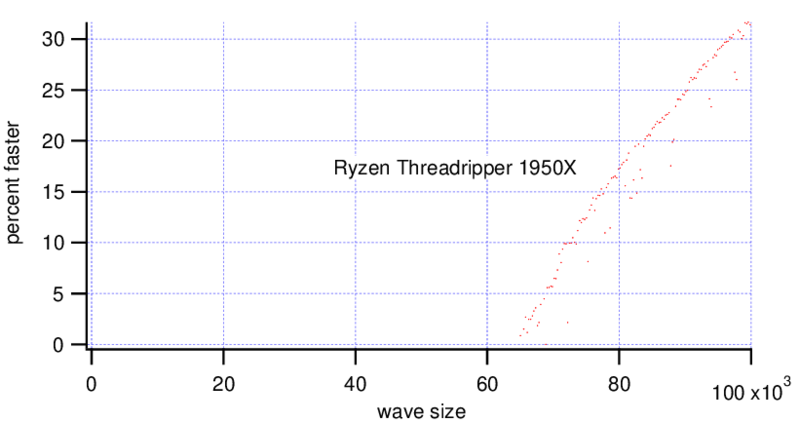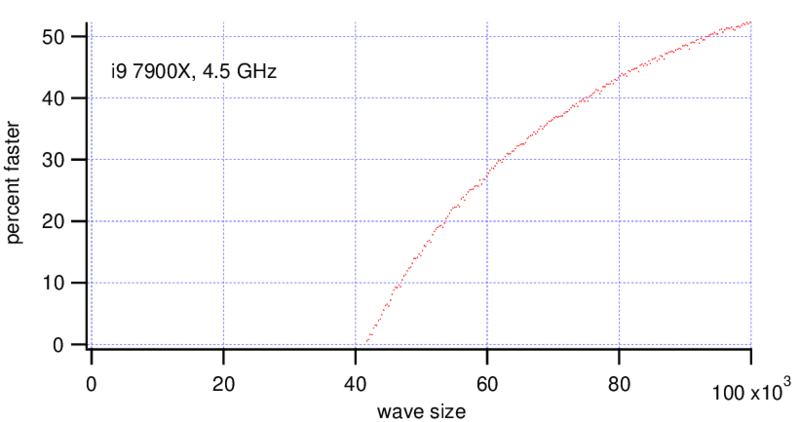MatrixOp is faster on the Threadripper with wave sizes bigger than ~70,000 points. On the i9 MatrixOp is faster for wave sizes bigger than ~40,000 points, at a wave size of 90,000 points it is already 2x faster.

• ##### data type double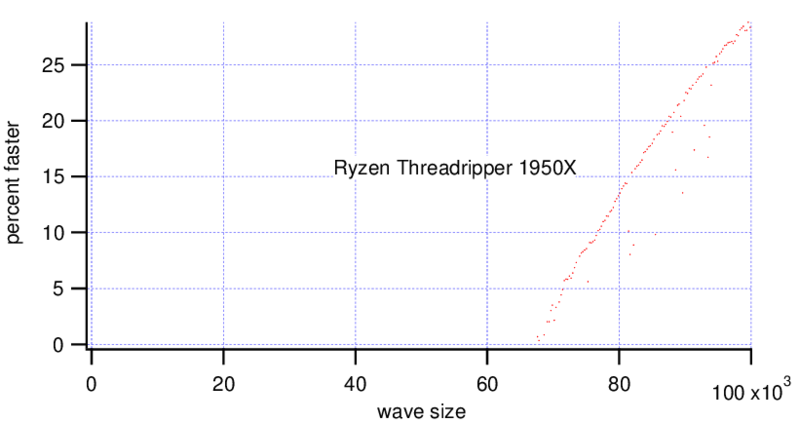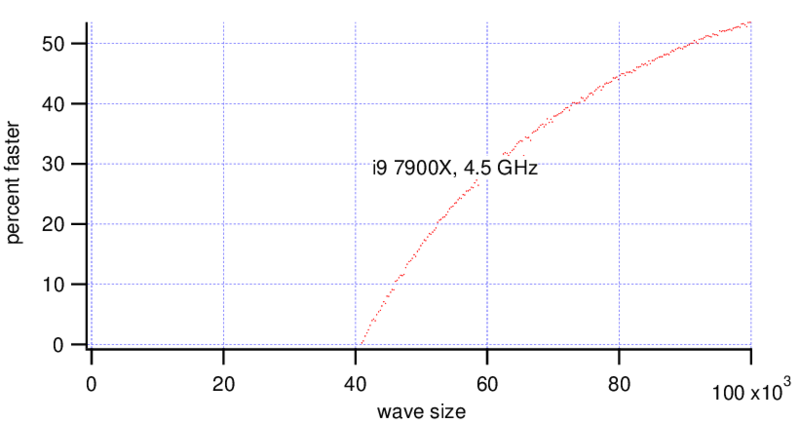Interestingly there is not much difference when changing the wave type to double precision.
MatrixOp is faster on the Threadripper with wave sizes bigger than ~70,000 points. On the i9 MatrixOp is faster for wave sizes bigger than ~40,000 points, at a wave size of 90,000 points it is already 2x faster.

• ##### data type byte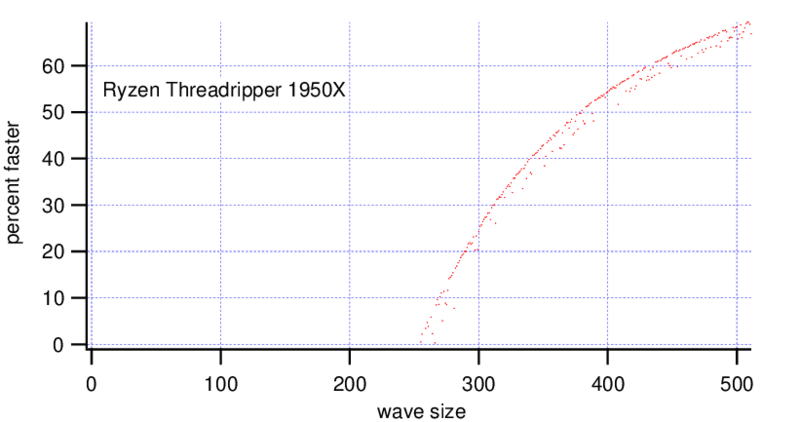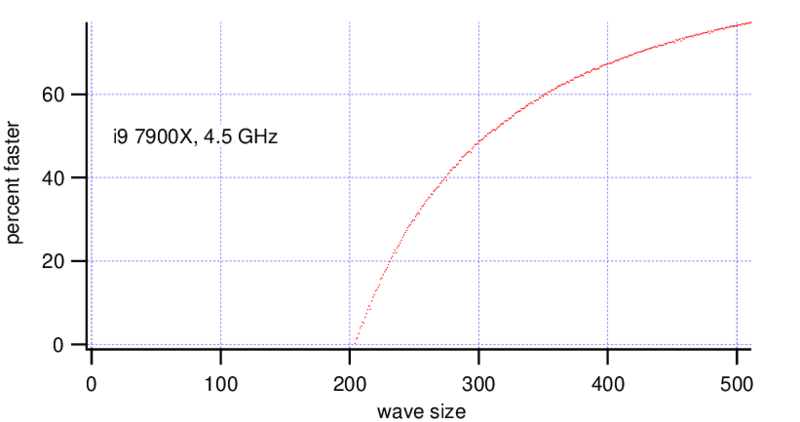MatrixOp is faster on the Threadripper with wave sizes bigger than ~(270, 270) points. On the i9 MatrixOp is faster for wave sizes bigger than (200, 200) points, at a wave size of (300, 300) points it is already 2x faster.

• ##### data type double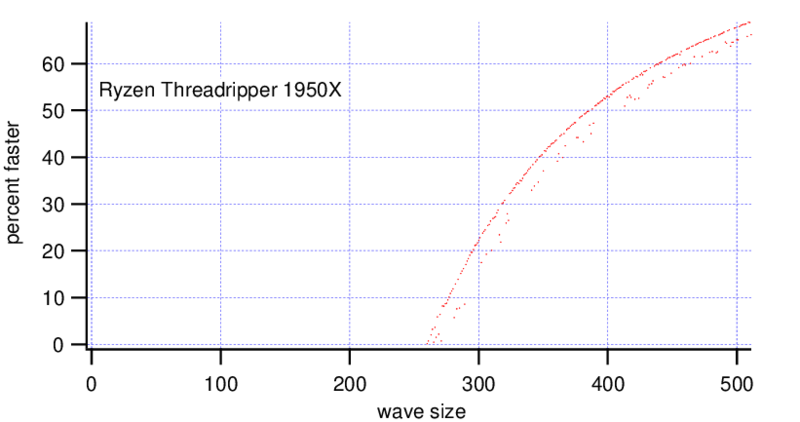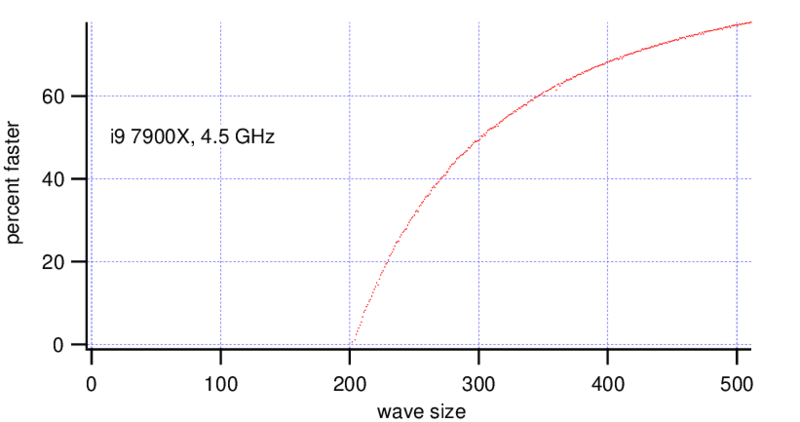Interestingly also for 2d waves there is not much difference if one uses byte or double type waves.
MatrixOp is faster on the Threadripper with wave sizes bigger than ~(270, 270) points. On the i9 MatrixOp is faster for wave sizes bigger than (200, 200) points, at a wave size of (300, 300) points it is already 2x faster.

For wave copy it turned out that it is only faster for 2D waves.

• ##### data type byte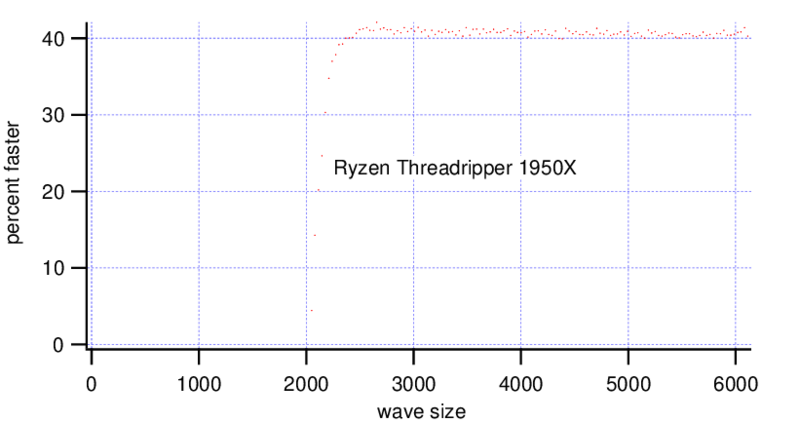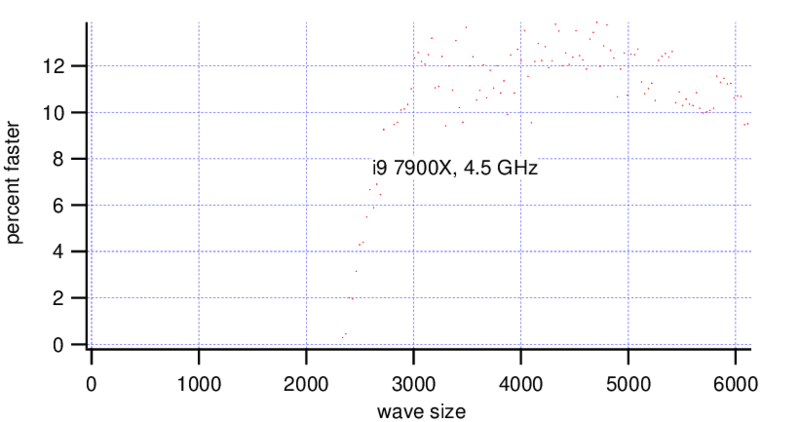Starting from a wave size of ~(2000, 2000) and bigger the Threadripper gets a consistent gain of 40%, so MatrixOp is nearly 2x faster than Duplicate.
The i9 shows only a a speed increase of ~10% for waves bigger than ~(2500, 2500).

• ##### data type double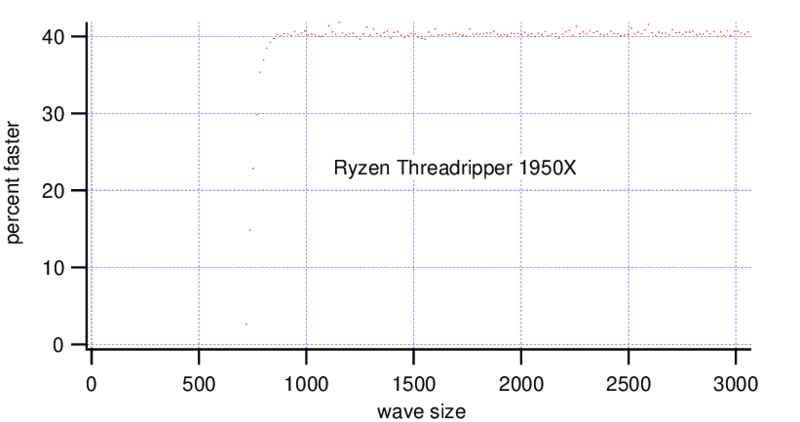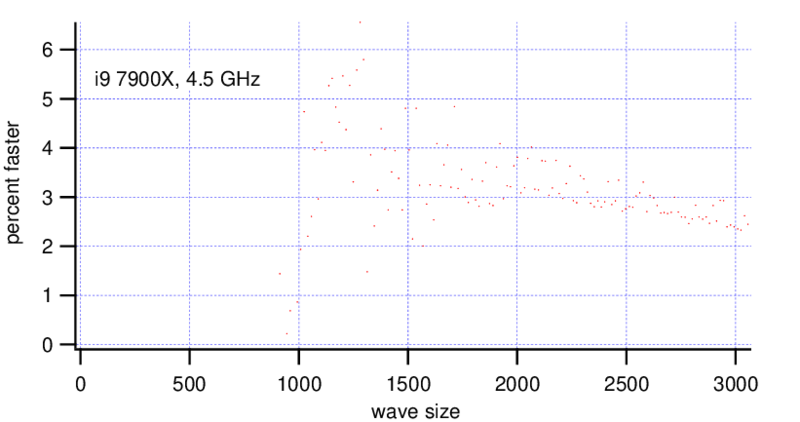For double precision waves the Threadripper shows a consistent speedup of 40% with waves bigger than ~(700, 700). The i9 shows a very slight gain for bigger waves.

#### Summary

The results show that with a recent CPU with mutiple cores the speed gain can be dramatical, when using the right approach. MatrixOp is one of the operations that uses automatically multithreading and can replace some common functions.
Especially when working with larger data sets a ~10x speedup is really good.

For wave copy of 1D waves it is worth considering to use a Redimension/E=1 to change the source wave to a 2D wave, then use MatrixOp and Redimension/E=1 the target wave back to 1D. (where possible).

Michael Huth
byte physics